# Formulation of photon diffusion from spherical bioluminescent sources in an infinite homogeneous medium

## Abstract

### Background

The bioluminescent enzyme firefly luciferase (Luc) or variants of green fluorescent protein (GFP) in transformed cells can be effectively used to reveal molecular and cellular features of neoplasia in vivo. Tumor cell growth and regression in response to various therapies can be evaluated by using bioluminescent imaging. In bioluminescent imaging, light propagates in highly scattering tissue, and the diffusion approximation is sufficiently accurate to predict the imaging signal around the biological tissue. The numerical solutions to the diffusion equation take large amounts of computational time, and the studies for its analytic solutions have attracted more attention in biomedical engineering applications.

### Methods

Biological tissue is a turbid medium that both scatters and absorbs photons. An accurate model for the propagation of photons through tissue can be adopted from transport theory, and its diffusion approximation is applied to predict the imaging signal around the biological tissue. The solution to the diffusion equation is formulated by the convolution between its Green's function and source term. The formulation of photon diffusion from spherical bioluminescent sources in an infinite homogeneous medium can be obtained to accelerate the forward simulation of bioluminescent phenomena.

### Results

The closed form solutions have been derived for the time-dependent diffusion equation and the steady-state diffusion equation with solid and hollow spherical sources in a homogeneous medium, respectively. Meanwhile, the relationship between solutions with a solid sphere source and ones with a surface sphere source is obtained.

### Conclusion

We have formulated solutions for the diffusion equation with solid and hollow spherical sources in an infinite homogeneous medium. These solutions have been verified by Monte Carlo simulation for use in biomedical optical imaging studies. The closed form solution is highly accurate and more computationally efficient in biomedical engineering applications. By using our analytic solutions for spherical sources, we can better predict bioluminescent signals and better understand both the potential for, and the limitations of, bioluminescent tomography in an idealized case. The formulas are particularly valuable for furthering the development of bioluminescent tomography.

## Background

Light propagation in scattering media has attracted attention in many areas of physics, biology, medicine, and engineering. This process can be described by the radiative transfer equation , which is a complex integrodifferential equation. Under strong scattering conditions, the radiative transfer equation can be reduced to the diffusion equation, but this still does not permit exact solutions in most cases. Recently, various approximate methods for the radiative transfer equation have been presented . Arridge et al.  presented a method for handling nonscattering regions within diffusing domains. The method develops from an iterative radiosity-diffusion approach that uses a finite element method. The fundamental idea is to introduce extra equations into the standard diffusion finite element method to represent nondiffusive light propagation across a nonscattering region. Aydin et al.  introduced a two-dimensional, finite element spherical harmonics radiation transport method for the simulation of light propagation in tissue. They derived a photon-transport forward model in turbid media that treats weak inhomogeneities through a Born approximation of the Boltzmann radiative transfer equation. This model more conveniently replaces the commonly used diffusion approximation in optical tomography . Analytic solutions to the diffusion equation in some simplified cases have been explored previously . Boas et al.  presented a spherical harmonic solution in the case of spherical inhomogeneity in an infinite medium. In this instance, a closed-form infinite series solution was obtained. Additionally, they compared their calculations to experimental results involving a perfect absorber in a large diffuse region. They observed good agreement between the experimental and the analytical data. Walker et al.  extended the above spherical results to cylindrical inhomogeneity. Fishkin et al.  derived analytical expressions based on the diffusion approximation that describe the photon density in a uniform, infinite, strongly scattering medium that contains a sinusoidally intensity-modulated point source of light. They also reported good agreement between their analytical and experimental results. However, these solutions to the diffusion equation were derived for a point source only.

Recently, light propagation in scattering media has attracted increased attention in biomedical applications , especially in bioluminescent imaging as applied in bioluminescent tomography. The bioluminescent enzyme firefly luciferase (Luc) or variants of green fluorescent protein (GFP) in transformed cells can be effectively used to reveal molecular and cellular features of neoplasia in vivo. Tumor cell growth and regression in response to various therapies can be evaluated by using bioluminescent imaging. With a number of key collaborators, we are developing a bioluminescent tomography system for the 3D reconstruction of a bioluminescent source distribution in a living animal marked by bioluminescent reporter luciferases from diffuse signals detected on a spherical surface containing the animal. In bioluminescent experiments, the density of the bioluminescent signals decay exponentially with time. Actually, bioluminescent imaging is a time-dependent problem. In this paper, we formulate solutions to the time-dependent diffusion equation and the steady-state diffusion equation with solid and hollow spherical sources, respectively, in an infinite homogeneous medium. These formulas are then verified in a Monte Carlo simulation performed using a commercial software package, TracePro. The solution to the diffusion model in a homogeneous medium can be used to achieve reasonably good qualitative estimates of the signal level and can be applied to estimate rapidly the positions of light sources for bioluminescent imaging. The closed form solution is highly accurate and more computationally efficient in biomedical engineering applications.

## Methods

The radiative transport equation in a media that scatters and absorbs photons is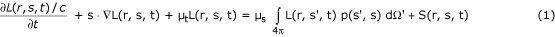L(r, s, t) is the radiance (the power per unit area and unit solid angle) at position r, traveling in direction s, at time t, in the unit of W/(m2•sr). C is the speed of light in the medium. μt = μs + μa is the optical transport coefficient, where μs is the scattering coefficient, and μa is the absorption coefficient. S(r, s, t) is the spatial and angular distribution of the source in the unit of W/(m3•sr). The normalized phase function p(s', s) represents the probability of scattering in a direction s' from direction s. The photon fluence is given by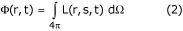The photon flux or current density is given by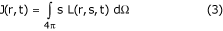Both the fluence and the flux are in units of W m-2. The transport equation can be regarded as a conservation equation for the radiance; it neglects coherence and polarization effects.

In vivo experimental light propagates in a highly scattering tissue in which the scattering coefficient is much greater than the absorption coefficient, and the diffusion approximation is sufficiently accurate. In the P1 approximation, the radiance is expanded in spherical harmonics truncated to the first order, and the radiance equation can be reduced to the diffusion equation [5, 9]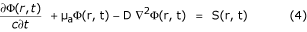where Φ(r, t) is the photon fluence rate; D = {3[μa + (1 - g)μs]}-1 is the diffusion coefficient; g is the anisotropy coefficient. Analytic solutions to the diffusion equation are difficult to obtain in most cases and its numerical solutions take large amounts of computational time. By setting S(r, t) = δ(r, t), the time-domain Green's function for an infinite homogeneous medium is given byThe Green's function (5) helps to further simplify the computation of the diffusion equation (4). The solution to the diffusion equation for a general source term S(r, t) is given by the convolution between S(r, t) and the Green's function Γ(r, t):In the following section, we analytically solve for the photon diffusion with spherical sources embedded in an infinite homogeneous medium.

## Solution for a spherical surface source

A spherical source with photon power uniformly distributed on the surface is defined bywhere p is the source power; R is the radius of the spherical source; and ω is the decay coefficient of the source power related to time.

In the spherical coordinates, the convolution integral (6) becomes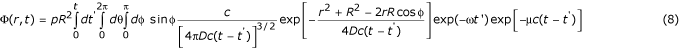Integrating with respect to the angular variables θ and φ, Eq. (8) becomes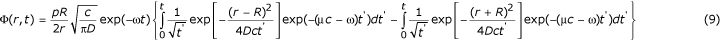Letting r ≥ R,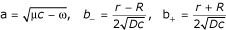, we have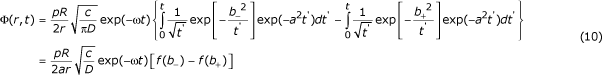where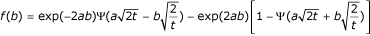, and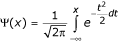.

## Solution for a spherical solid source

A spherical solid source with a radially and exponentially decreasing power density is defined by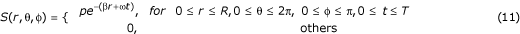where p is the source power; R is the radius of the spherical source; ω is the decay coefficient of the source power related to time; and β is the exponential decay coefficient related to the radius of the spherical source.

The convolution integral (6) becomesIntegrating with respect to the angular variables θ and φ, and changing the order of integration, Eq. (12) becomes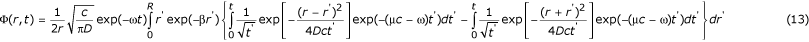Setting r ≥ R,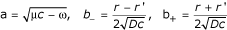, Φ(r, t) can be expressed as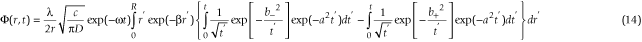Performing an integration around variable t', we obtain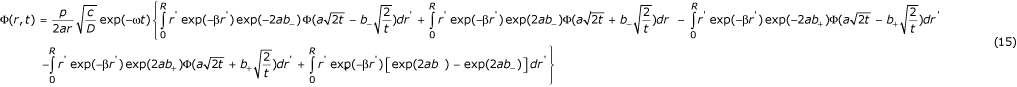Integrating with respect to variables r', we obtainwhere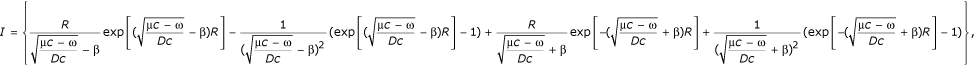and E(R, k, a, b) is defined asSetting β = 0, we obtain the solution for a spherical solid source with a constant power density as follows: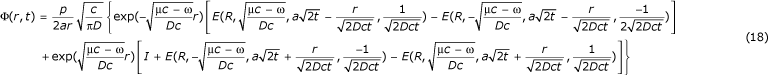where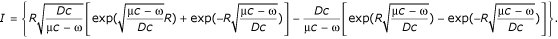## Solutions to the steady-state diffusion equation

Similarly, expressing the solution of the steady-state diffusion equation as the convolution between S(r, t) and its Green's function, and simplifying the convolution, the solutions to the steady-state diffusion equation can be easily derived for spherical surface and solid sources, respectively. In this case, the solution for a spherical surface source can be shown to be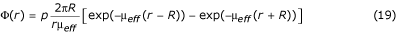and the solution for a spherical solid source can be expressed aswhere p is the source power; R is the radius of the spherical source; and μeff denotes the effective attenuation coefficient defined as μeff = (μa /D)1/2 = [3 μaa + μs (1 - g))]1/2.

## Results

To verify the correctness of the above analytic results, two sets of computer simulation experiments were performed using the well-known MatLab and a commercial software package, TracePro (Lambda Research Corp., Littleton, MA, USA; http://www.lambdares.com/products/tracepro/index.phtml). The first set of tests examined the relationship between the solutions for spherical surface and solid sources. The other set of tests demonstrated good agreement between the analytically computed and the Monte Carlo simulated data.

## Comparison between solutions for spherical solid and surface sources

The numerical tests were conducted with various parameters using solutions for spherical surface and solid sources. In the first experimental setting, a spherical solid source of radius 1 mm with power 0.0002513 (W) was embedded in an infinite homogeneous medium. The medium was defined by μs = 40.3 mm-1, μa = 0.15 mm-1, n = 1, and g = 0.994. In the second setting, a spherical solid source of radius 0.3 mm with power 0.0002513 (W) was assumed, with a medium of μs = 10.27 mm-1, μa = 0.082 mm-1, n = 1.37, and g = 0.96. Figs. 1 and 2 present some typical analytically calculated outcomes. These results indicate that the directly computed data using the solution formula for a spherical solid source are in an excellent agreement with their counterparts indirectly computed using the solution for a spherical surface source. In other words, the two solutions are consistent.

## Comparison between analytic solution and Monte Carlo simulation

In the following two numerical experimental settings, the optical parameters of the medium were set at μs = 23 mm-1, μa = 0.35 mm-1, n = 1, and g = 0.94, the same as the optical parameters of a mouse lung. The source, a sphere with a radius of 1.3 mm and power 0.00025 (W), was embedded in an infinite homogeneous medium. The power incidence on the spherical surfaces with different radii was computed by using the solution formulas and the Monte Carlo method as implemented in TracePro, respectively. Comparison of the two is shown in Fig. 3. In the other numerical experiment, the optical parameters of the medium were μs = 16 mm-1, μa = 0.20 mm-1, n = 1.37, and g = 0.85, the same as the optical parameters of a mouse heart. The source is a sphere of radius 1 mm with power 0.00025 (W). Fig. 4 presents a comparison of the analytic results and the simulated data from TracePro. It can be observed that there is good agreement between the computed and the simulated data.

## Discussions and conclusion

The primary utility of our analytic solutions is to accelerate the forward simulation of bioluminescent phenomena which will facilitate studies on bioluminescent tomography. The spherical sources we assumed are the most fundamental building blocks of the physical phantoms used in the evaluation of image quality. By using our analytic solutions for spherical sources, we can better predict bioluminescent signals and better understand both the potential for, and the limitations of, bioluminescent tomography in an idealized case.

In conclusion, we have formulated solutions for the diffusion equation with solid and hollow spherical sources in an infinite homogeneous medium. These solutions have been verified by Monte Carlo simulation for use in biomedical optical imaging studies. The formulas are particularly valuable for furthering the development of bioluminescent tomography.

## APPENDIX: Integral formulas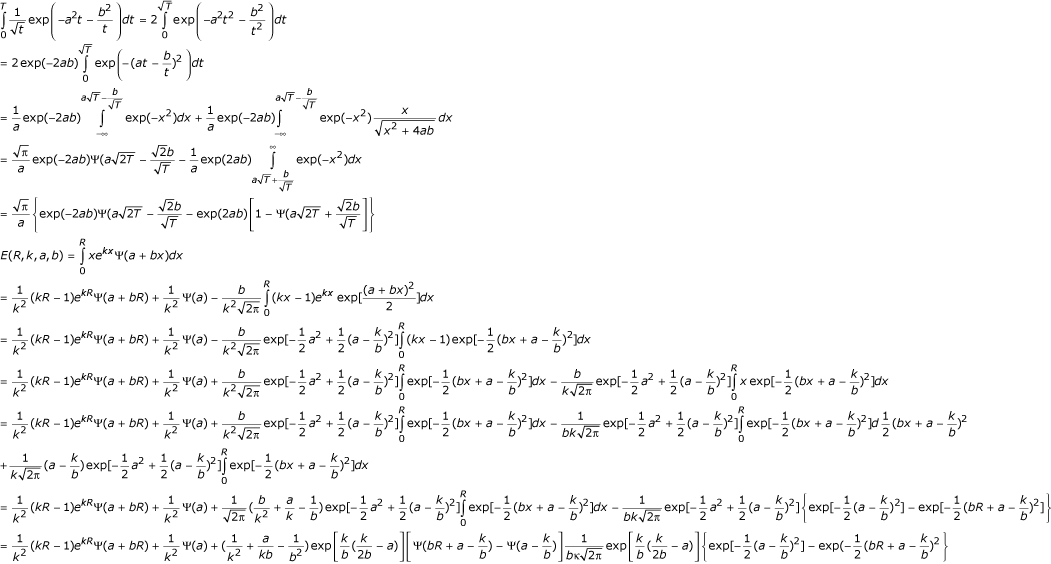## References

1. Case KM, Zweifel PF: Linear Transport Theory. Mass: Addison-Wesley 1967.

2. Arridge SR, Deghani H, Schweiger M, Okada E: The finite element model for the propagation of light in scattering media: a direct method for domains with nonscattering regions. Med Phys 2000, 27: 252–264. 10.1118/1.598868

3. Aydin ED, Oliveira CR, Goddard AJ: A comparison between transport and diffusion calculations using a finite element-spherical harmonicsradiation transport method. Med Phys 2002, 29: 2013–2023. 10.1118/1.1500404

4. Xu M, Cai W, Lax M, Alfano RR: Photon transport forward model for imaging in turbid media. Opt Lett 2001, 26: 1066–1068.

5. Boas DA: Diffuse Photon Probes of Structural and Dynamical Properties of Turbid Media, PHD. Thesis, University of Pennsylvania, Philadelphia 1996.

6. Walker SA, Boas DA, Gratton E: Photon density waves scattered from cylindrical inhomogeneities: theory and experiments. Appl Opt 1998, 37: 1935–1944.

7. Fishkin JB, Gratton E: Propagation of photon-density waves in strongly scattering media containing an absorbing semi-infinite plane bounded by a straight edge. J Opt Soc Am A 1993, 10: 127–140.

8. Rice BW, Cable MD, Nelson MB: In vivo imaging of light-emitting probes. J of Bio Opt 2001, 6: 432–440. 10.1117/1.1413210

9. Arridge SR, Schweiger M, Hiraoka M, Delpy DT: A finite element approach for modeling photon transport in tissue. Med Phys 1993, 20: 299–309. 10.1118/1.597069

10. Wang LH, Jacques SL, Zheng LQ: MCML-Monte Carlo modeling of photon transport in multilayered tissues. Comput Methods Programs Biomed 1995, 47: 131–146. 10.1016/0169-2607(95)01640-F

11. Patterson MS, Chance B, Wilson BC: Time resolved reflectance and transmittance for the non-invasive measurement of optical properties. Appl Opt 1989, 28: 2331–2336.

12. Markel VA, Schotland JC: Inverse problem in optical diffusion tomography. 1 Fourier-Laplace inversion formulas. J Opt Soc Am A Opt Image Sci Vis 2001, 18: 1336–1347.

13. Haskell RC, Svaasand LO, Tsay T, Feng T, McAdams MS, Tromberg BJ: Boundary conditions for the diffusion equation in radiative transfer. J Opt Soc Am A 1994, 11: 2727–2741.

14. Flock ST, Patterson MS, Wilson BC, Wyman DR: Monte Carlo modeling of light propagation in highly scattering tissues- 1: Model predictions and comparison with diffusion theory. IEEE Trans Biomed Eng 1989, 36: 1162–1168. 10.1109/TBME.1989.1173624

15. Sassaroli A, Blumetti C, Martelli F, Alianelli L, Contini D, Ismaelli A, Zaccanti G: Monte Carlo procedure for investigating light propagation and imaging of highly scattering media. Appl Opt 1998, 37: 7392–7400.

## Acknowledgment

This work was supported in part by the NIH grant R21/R33 EB001685.

## Author information

Authors

### Corresponding author

Correspondence to Wenxiang Cong.

## Authors’ original submitted files for images

Below are the links to the authors’ original submitted files for images.

## Rights and permissions

Reprints and Permissions

Cong, W., Wang, L.V. & Wang, G. Formulation of photon diffusion from spherical bioluminescent sources in an infinite homogeneous medium. BioMed Eng OnLine 3, 12 (2004). https://doi.org/10.1186/1475-925X-3-12

• Accepted:

• Published:

• DOI: https://doi.org/10.1186/1475-925X-3-12

### Keywords

• Diffusion equation
• Green's function
• analytical solution
• Monte Carlo simulation
• bioluminescent imaging